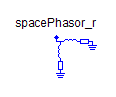M_PMOS2 - MapleSim Help

M_PMOS2

PMOS MOSFET deviceDescription The M_PMOS2 component models a P-channel MOSFET transistor using a level 2 Schichman-Hodges model. The model card parameter specifies an M2_Card record that contains the common technology parameters for the class of mosfets.Connections

 Name Description Modelica ID $G$ gate node G $\mathrm{D}$ drain node D $S$ source node S $B$ bulk node BParameters

 Name Default Units Description Modelica ID $L$ $1·{10}^{-4}$ $m$ Length L $W$ $1·{10}^{-4}$ $m$ Width W ${A}_{\mathrm{D}}$ $0$ ${m}^{2}$ Area of the drain diffusion AD ${A}_{S}$ $0$ ${m}^{2}$ Area of the source diffusion AS ${P}_{\mathrm{D}}$ $0$ $m$ Perimeter of the drain junction PD ${P}_{S}$ $0$ $m$ Perimeter of the source junction PS ${\mathrm{NR}}_{\mathrm{D}}$ $1$ Number of squares of the drain diffusions NRD ${\mathrm{NR}}_{S}$ $1$ Number of squares of the source diffusions NRS ${\mathrm{IC}}_{\mathrm{VDS}}$ $-1·{10}^{40}$ $V$ Initial condition value (VDS, not implemented yet) IC_VDS ${\mathrm{IC}}_{\mathrm{VGS}}$ $-1·{10}^{40}$ $V$ Initial condition value (VGS, not implemented yet) IC_VGS ${\mathrm{IC}}_{\mathrm{VBS}}$ $-1·{10}^{40}$ $V$ Initial condition value (VBS, not implemented yet) IC_VBS $\mathrm{UIC}$ $\mathrm{false}$ Use initial conditions: true, if initial condition is used UIC T $27$ $\mathrm{°C}$ Operating temperature of the device TEMP model card MOSFET modelcard modelcardModelica Standard Library The component described in this topic is from the Modelica Standard Library. To view the original documentation, which includes author and copyright information, click here.